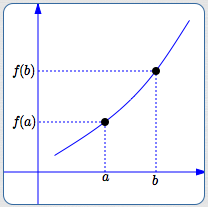﻿ Increasing and Decreasing Functions

# INCREASING AND DECREASING FUNCTIONS

• PRACTICE (online exercises and printable worksheets)

## DEFINITIONS:

 A function $\,f\,$ increases on an interval $\,I\,$ if and only if whenever $\,a < b\,$ in $\,I\,$, $\,f(a) < f(b)\,$.A function $\,f\,$ decreases on an interval $\,I\,$ if and only if whenever $\,a < b\,$ in $\,I\,$, $\,f(a) > f(b)\,$.Roughly:
increasing means going strictly UPHILL, as you move from left to right on a graph
decreasing means going strictly DOWNHILL, as you move from left to right on a graph
Master the ideas from this section